IF YOU CAN SEE THIS go to /sfa/Nuprl/Shared/Xindentation_hack_doc.html

### Atoms

"abc", "123", "d &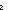"
These are samples of expressions that we consider, whenever they are used, to be denoting discrete atomic entities, despite the fact that we can see characters constituting the expressions. The use of such characters permits some mnemonic exploitation.
However, there are no constructors or destructors in the theory for these atoms, nor is there a "new atom" generator. There is only a method for checking identity. If one uniformly replaced all the atom constants throughout a statement in Nuprl, preserving identity and difference, the result would still be true (or false) if the original was.

"if a=b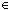Atom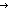uv fi" compares such atomic expressions a and b and continues by executing expression u or v accordingly.

if "abc"="abc"Atom1; 2 fi *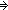1

if "abc"="def"Atom1; 2 fi *2

There is also an often-used Boolean expression for atom equality:

Def x=yAtom == if x=yAtomtrue; falsefi

Thm*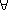x,y:Atom. x=yAtom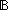Atom is the type of such atoms.

It's a bit subtle, in theory, but natural in practice.

There is no assumption pertaining to finiteness. One cannot prove that the type is finite or that it is not. However, for any number one can prove there are at least that many members of Atom, simply by showing that many examples chosen at will. This is odd.

Perhaps the best interpretation of Atom is to consider it not as a specific type, but simply as a variable of the semantics that, given a body of discourse, can be interpreted as any discrete type (i.e., a type with decidable equality) having at least as many elements as there are distinct atom constants actually used in the given body of discourse.

Another possible interpretation is as a primitive open-ended type whose members are introduced one-by-one as needed. Thus, one can always find a new member, but there's no systematic function for producing it. This seems as sensible as the intuitionistic notion of free choice sequence.

(Feb 2001 - sfa )